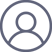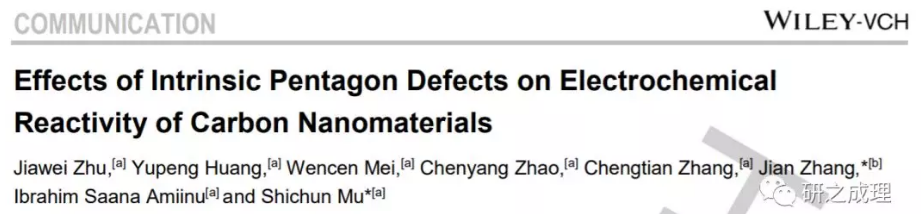木士春2019-2-7 22:38

## 原创： 木士春教授课题组 研之成理 2019.02.07A.    碳纳米材料的电化学应用

B.    缺陷工程调控

C.    研究的出发点Figure 1. HOMO and LUMO distributions of (a, b) C6 and (c, d) C5; charge densities of (e) C6 and (f) C5; (g) oxygen adsorption energies of C6 and C5.

A.    催化剂合成Figure 2. (a) Schematic illustration of the synthetic method of PD-C; (b) ac-STEM image of PD-C; (c) cropped image of b; (d) XRD patterns, (e) Raman spectra, (f) N2 adsorption-desorption isotherms and corresponding pore size distribution curves of PD-C and C60; (g) C1s fitting results of PD-C.

B.    电化学表征Figure 3. ORR tests in 0.1 M KOH. (a) LSV curves of G, D-G, C60 and PD-C catalysts; LSV curves at different rotating rates and inserted K-L plots of (b) PD-C and (c) D-G; (d) i-t chronoamperometric responses, (e) methanol tolerance tests and (f) carbon monoxide resistance tests of PD-C and D-G.

C.    进一步的性能优化Figure 4. (a) Schematic diagram of bifunctional PD/N-C material; (b) LSV curves of PD/N-C and commercial Pt/C catalyst in 0.1 M KOH; (c) polarization curves and power density curves of the Zn-air batteries with PD/N-C and Pt/C cathodes; (d) assembly diagram of all-solid-state Zn-air battery with the digital photographs of OCP test and luminous LED lamp beads; (e) galvanostatic charge-discharge curves at 1A g-1 and (f) rate capability of PD/N-C and PD-C; (g) cycling stability of PD/N-C at 10A g-1 (inset: electrochemical impedance spectroscopy plots of PD/N-C and PD-C).